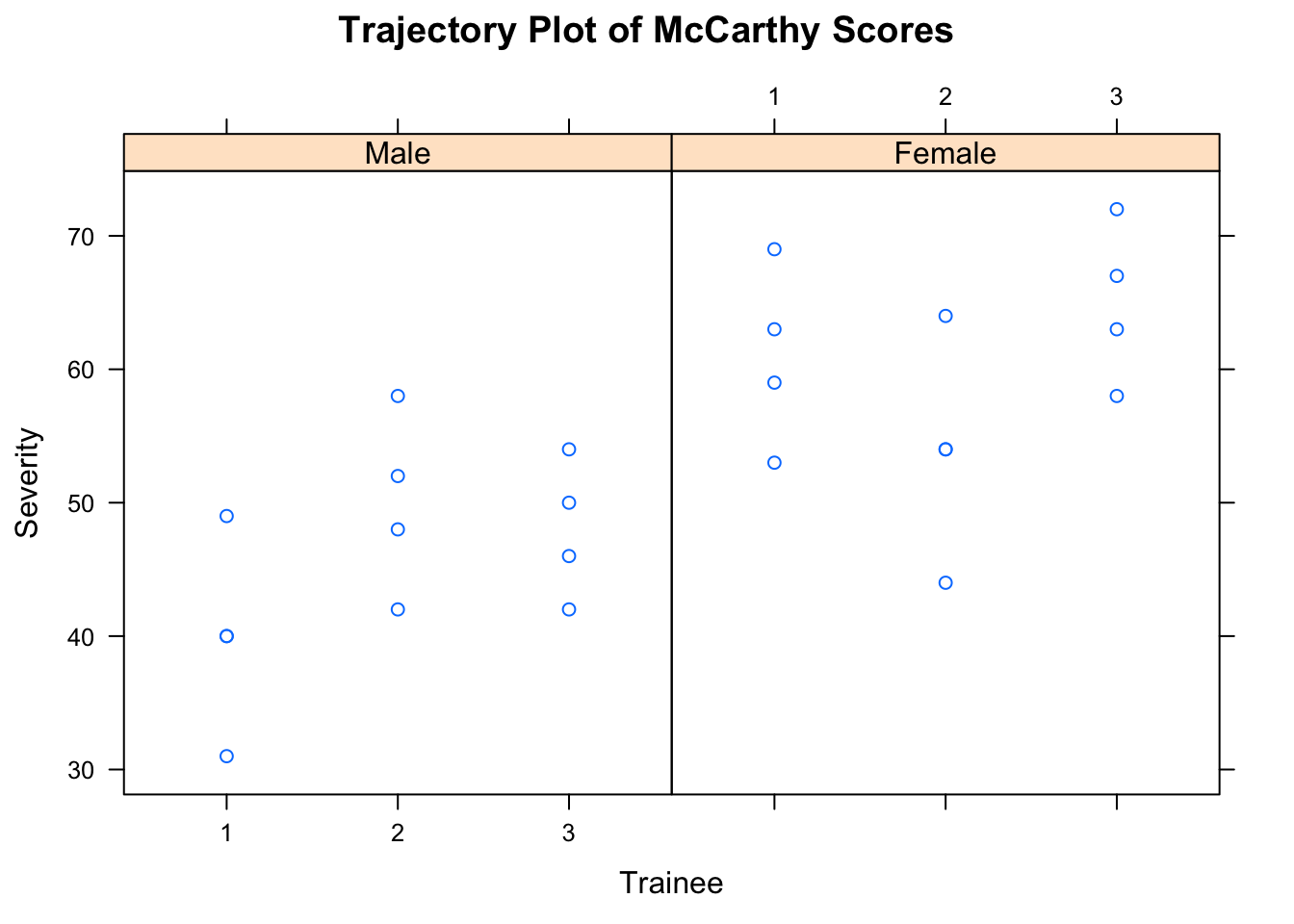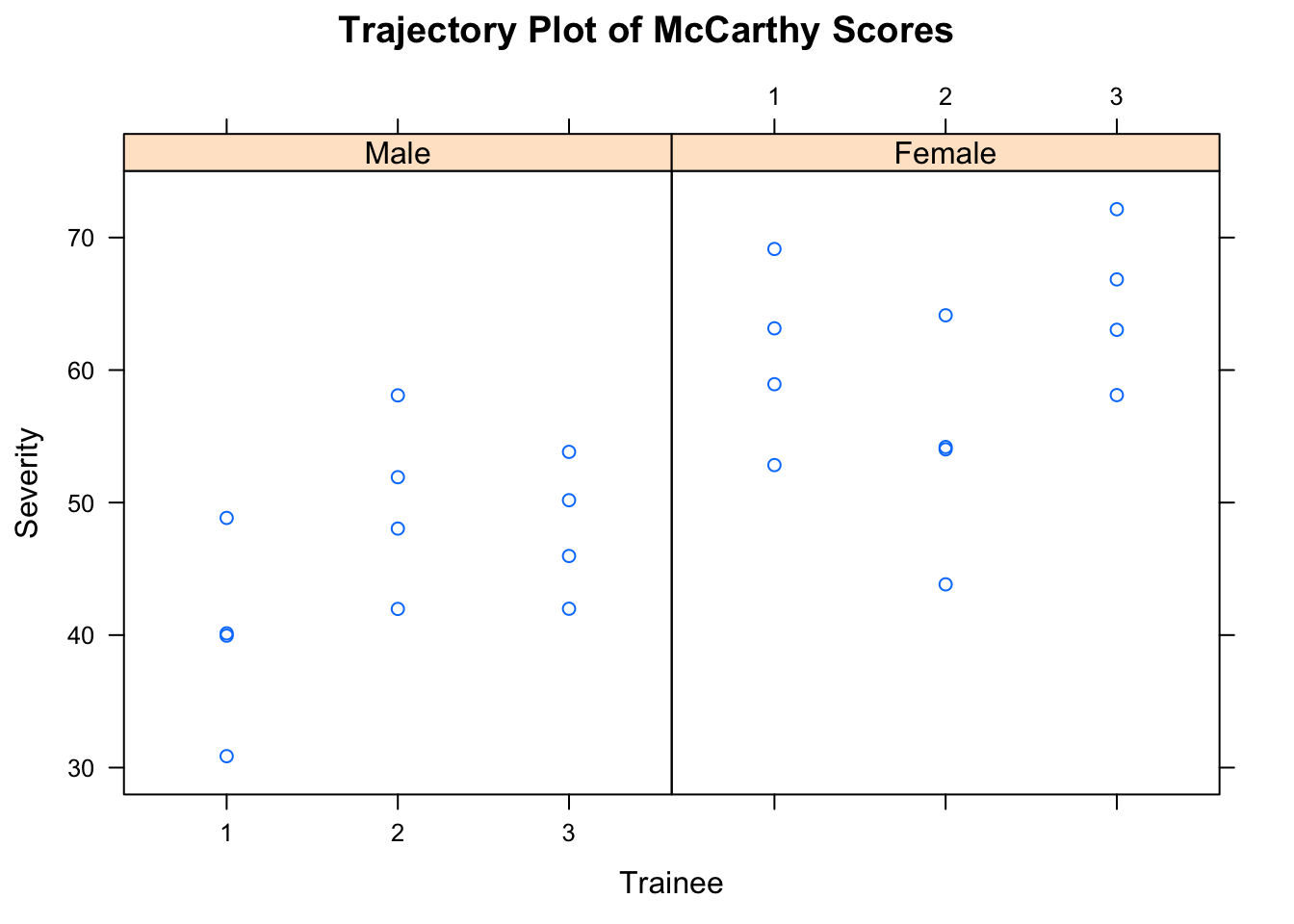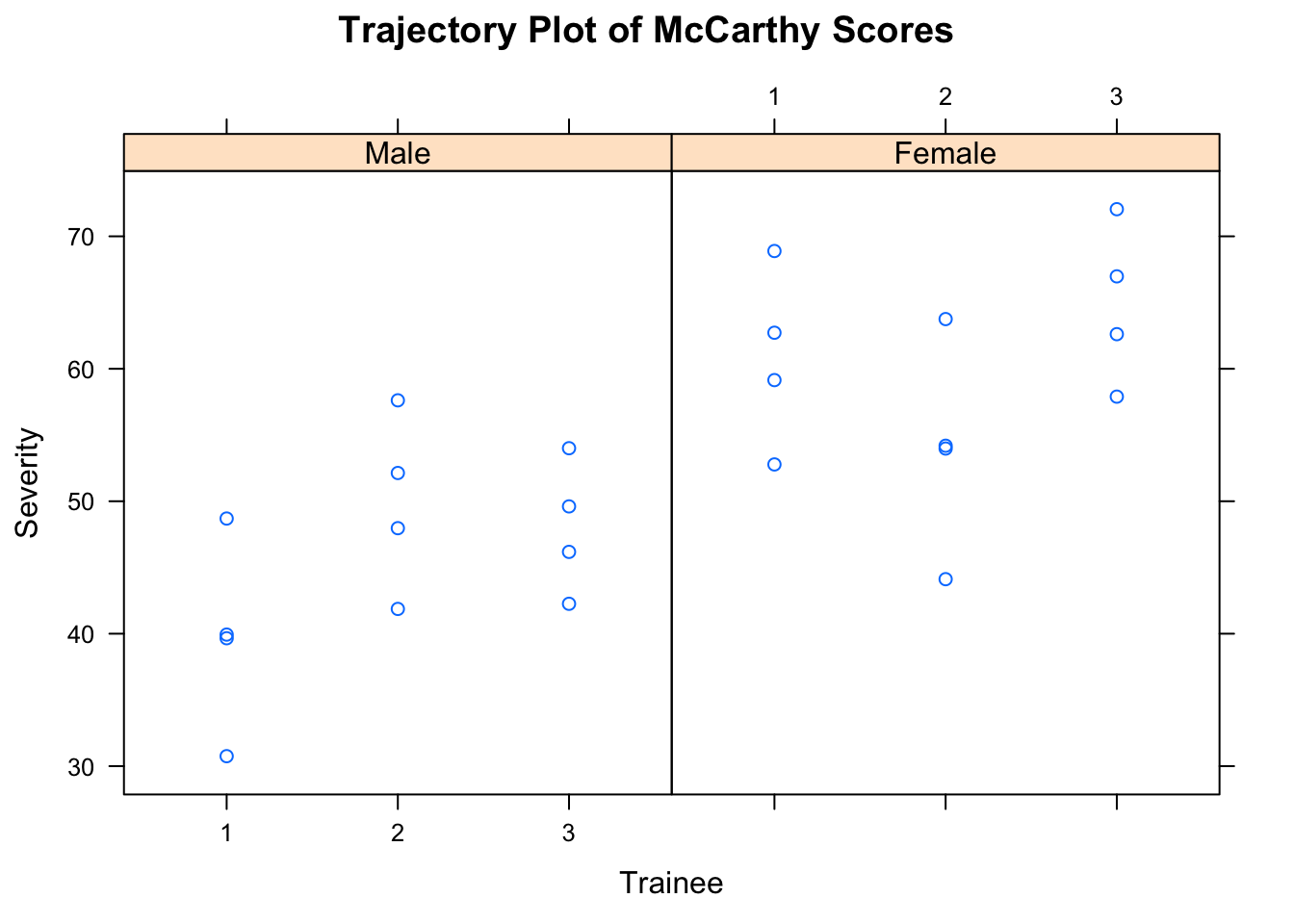# Designing Experiments and Analyzing Data: A Model Comparison Perspective (3rd edition) by Maxwell, Delaney, & Kelley

## Information about the book is available at https://designingexperiments.com

### R Code and Instructions to Accompany Chapter 16

These notes build on the prior chapters’ R Code and Instructions, where we discuss some operational aspects of R and detail the use of many functions and how to install packages. Here, we skip details that have already been discussed in the prior R Code and Instructions.

Load the AMCP package to use the data from Table 1, Chapter 16.

``````library(AMCP)
data(chapter_16_table_1)
chapter_16_table_1``````
``````##    Severity Trainee Gender
## 1        49       1      1
## 2        40       1      1
## 3        31       1      1
## 4        40       1      1
## 5        42       2      1
## 6        48       2      1
## 7        52       2      1
## 8        58       2      1
## 9        42       3      1
## 10       46       3      1
## 11       50       3      1
## 12       54       3      1
## 13       53       4      2
## 14       59       4      2
## 15       63       4      2
## 16       69       4      2
## 17       44       5      2
## 18       54       5      2
## 19       54       5      2
## 20       64       5      2
## 21       58       6      2
## 22       63       6      2
## 23       67       6      2
## 24       72       6      2``````

Get the group descriptive statistics (this is one appraoch; see the Chapter 4 R Accompaniment for other approaches).

``library(dplyr)``
``````##
## Attaching package: 'dplyr'``````
``````## The following objects are masked from 'package:stats':
##
##     filter, lag``````
``````## The following objects are masked from 'package:base':
##
##     intersect, setdiff, setequal, union``````
``````Summary.16.1 <- chapter_16_table_1 %>%
group_by(Gender) %>%
summarize(
mean=mean(Severity),
sd=sd(Severity),
s2=var(Severity),
n=n()
)
Summary.16.1``````
``````## # A tibble: 2 × 5
##   Gender  mean       sd       s2     n
##    <int> <dbl>    <dbl>    <dbl> <int>
## 1      1    46 7.397788 54.72727    12
## 2      2    60 7.920055 62.72727    12``````

It may be helpful to extend the above so that descriptives are provided for the trainees within the groups. We call the object created here `Summary.16.1b` to distinguish it from the above summary.

``````Summary.16.1b <- chapter_16_table_1 %>%
group_by(Gender,Trainee) %>%
summarize(
mean=mean(Severity),
sd=sd(Severity),
s2=var(Severity),
n=n()
)
Summary.16.1b``````
``````## Source: local data frame [6 x 6]
## Groups: Gender [?]
##
##   Gender Trainee  mean       sd       s2     n
##    <int>   <int> <dbl>    <dbl>    <dbl> <int>
## 1      1       1    40 7.348469 54.00000     4
## 2      1       2    50 6.733003 45.33333     4
## 3      1       3    48 5.163978 26.66667     4
## 4      2       4    61 6.733003 45.33333     4
## 5      2       5    54 8.164966 66.66667     4
## 6      2       6    65 5.944185 35.33333     4``````

Although not necessary, to be consistent with the book we modify the Trainee numbers. Trainee numbers 4, 5, and 6 in the data here corresponds to what is female Trainees 1, 2, and 3 in the book. The code below extracts the Trainee numbers for `Gender=1` and uses those as is, but for `Gender=2` it subtracts 3, so as to start numbering within female at 1. We create a new variable named `train` so as to maintain the integrity of the original data set.

``````chapter_16_table_1\$Trainee <- c(chapter_16_table_1\$Trainee[chapter_16_table_1\$Gender==1], chapter_16_table_1\$Trainee[chapter_16_table_1\$Gender==2]-3)
chapter_16_table_1``````
``````##    Severity Trainee Gender
## 1        49       1      1
## 2        40       1      1
## 3        31       1      1
## 4        40       1      1
## 5        42       2      1
## 6        48       2      1
## 7        52       2      1
## 8        58       2      1
## 9        42       3      1
## 10       46       3      1
## 11       50       3      1
## 12       54       3      1
## 13       53       1      2
## 14       59       1      2
## 15       63       1      2
## 16       69       1      2
## 17       44       2      2
## 18       54       2      2
## 19       54       2      2
## 20       64       2      2
## 21       58       3      2
## 22       63       3      2
## 23       67       3      2
## 24       72       3      2``````

For purposes of having a unique identification value for each participant, we create an `ID` variable by pasting Gender and Trainee number. We also add `G_` and `T_` to the Gender and Trainee numbers, respectively, to serve an an identifier.

``````chapter_16_table_1\$ID <- paste("G=", chapter_16_table_1\$Gender, "_", "T=",
chapter_16_table_1\$Trainee, sep="")
chapter_16_table_1``````
``````##    Severity Trainee Gender      ID
## 1        49       1      1 G=1_T=1
## 2        40       1      1 G=1_T=1
## 3        31       1      1 G=1_T=1
## 4        40       1      1 G=1_T=1
## 5        42       2      1 G=1_T=2
## 6        48       2      1 G=1_T=2
## 7        52       2      1 G=1_T=2
## 8        58       2      1 G=1_T=2
## 9        42       3      1 G=1_T=3
## 10       46       3      1 G=1_T=3
## 11       50       3      1 G=1_T=3
## 12       54       3      1 G=1_T=3
## 13       53       1      2 G=2_T=1
## 14       59       1      2 G=2_T=1
## 15       63       1      2 G=2_T=1
## 16       69       1      2 G=2_T=1
## 17       44       2      2 G=2_T=2
## 18       54       2      2 G=2_T=2
## 19       54       2      2 G=2_T=2
## 20       64       2      2 G=2_T=2
## 21       58       3      2 G=2_T=3
## 22       63       3      2 G=2_T=3
## 23       67       3      2 G=2_T=3
## 24       72       3      2 G=2_T=3``````

There are multiple ways of producing plots in the context of nested data structures. One convienient way is using the `xyplot()` function from the lattice package. The lattice package provides a great deal of flexibility.

``````library(lattice)
# (each ID has it's own plot)
xyplot(Severity ~ as.factor(Trainee) | factor(Gender, labels=c("Male", "Female")),
data=chapter_16_table_1, main="Trajectory Plot of McCarthy Scores", ylab="Severity", xlab="Trainee")````````````# Include jitter in order for different values to help differentiate points.
xyplot(jitter(Severity) ~ as.factor(Trainee) | factor(Gender, labels=c("Male", "Female")),
data=chapter_16_table_1, main="Trajectory Plot of McCarthy Scores", ylab="Severity", xlab="Trainee")````````````# Here we increase the amount of jittering.
xyplot(jitter(Severity, factor=2) ~ as.factor(Trainee) | factor(Gender, labels=c("Male", "Female")),
data=chapter_16_table_1, main="Trajectory Plot of McCarthy Scores", ylab="Severity", xlab="Trainee")``````As discussed for Chapter 15, the lme4 package is a widely used package for mixed-effects models, or what we is often termed multilevel models or hierarchical linear models, in R. Notice, below, that the fixed effects are written in the same way that the `lm()` function is used for linear models, whereas the random effects are included in parentheses with a pipe symbol (i.e., “|”) used to denote the nesting strucutre. The “(1|ID)” part, in words, means that “the intercept is nested within ID,” which means that there is a random intercept for each clustering variable. The model below models the overall mean value (intercept) and the variation around that mean (variance of the intercept). Note that the intercept is incuded by default in the fixed part of the model specification.

``library(lme4)``
``## Loading required package: Matrix``
``````Model.0 <- lmer(Severity ~ 1 + (1|ID), data=chapter_16_table_1)
summary(Model.0)``````
``````## Linear mixed model fit by REML ['lmerMod']
## Formula: Severity ~ 1 + (1 | ID)
##    Data: chapter_16_table_1
##
## REML criterion at convergence: 166.2
##
## Scaled residuals:
##      Min       1Q   Median       3Q      Max
## -1.59965 -0.49688 -0.01505  0.60318  1.50207
##
## Random effects:
##  Groups   Name        Variance Std.Dev.
##  ID       (Intercept) 71.01    8.427
##  Residual             45.56    6.749
## Number of obs: 24, groups:  ID, 6
##
## Fixed effects:
##             Estimate Std. Error t value
## (Intercept)   53.000      3.706    14.3``````

Here we include Gender as a predictor, which, because it is represented by a number, should have `as.factor()` added (or be specified as a factor in the data frame). Notice here that the SAS output given in the book uses a `Gender=2` for the reference groups, whereas R uses `Gender=1`. Thus, the intercept in the book of 60 is the model implied value for `Gender=2`, whereas the intercept given by the code below is 46 for `Gender=1`. However, because the “effect” of Gender is 14 units (-14 when `Gender=2` is the reference and 14 when `Gender=1` is the reference), the models are equivalent. Compare this with the output of Table 16.3.

``````Model.1 <- lmer(Severity ~ 1 + as.factor(Gender) + (1|ID), data=chapter_16_table_1)
summary(Model.1)``````
``````## Linear mixed model fit by REML ['lmerMod']
## Formula: Severity ~ 1 + as.factor(Gender) + (1 | ID)
##    Data: chapter_16_table_1
##
## REML criterion at convergence: 155.2
##
## Scaled residuals:
##     Min      1Q  Median      3Q     Max
## -1.8248 -0.4452 -0.1247  0.6843  1.4141
##
## Random effects:
##  Groups   Name        Variance Std.Dev.
##  ID       (Intercept) 18.11    4.256
##  Residual             45.56    6.749
## Number of obs: 24, groups:  ID, 6
##
## Fixed effects:
##                    Estimate Std. Error t value
## (Intercept)          46.000      3.136  14.669
## as.factor(Gender)2   14.000      4.435   3.157
##
## Correlation of Fixed Effects:
##             (Intr)
## as.fctr(G)2 -0.707``````

# We now move to the Inductive Reasoning Study.

Load the data into the workspace.

``````data(chapter_16_table_4)
chapter_16_table_4``````
``````##    Observation Room Condition Cognition Skill Inductive
## 1            1    1         0        46     4        21
## 2            2    1         0        52     4        26
## 3            3    1         0        60     4        33
## 4            4    1         0        44     4        22
## 5            5    2         0        46     6        18
## 6            6    2         0        48     6        25
## 7            7    2         0        50     6        26
## 8            8    2         0        54     6        24
## 9            9    2         0        50     6        21
## 10          10    2         0        48     6        25
## 11          11    3         0        52     9        35
## 12          12    3         0        50     9        28
## 13          13    3         0        46     9        32
## 14          14    3         0        50     9        36
## 15          15    3         0        58     9        38
## 16          16    1         1        42     5        26
## 17          17    1         1        46     5        34
## 18          18    1         1        50     5        27
## 19          19    2         1        52     8        38
## 20          20    2         1        54     8        44
## 21          21    2         1        46     8        34
## 22          22    2         1        56     8        45
## 23          23    2         1        48     8        38
## 24          24    3         1        42     7        31
## 25          25    3         1        46     7        41
## 26          26    3         1        44     7        34
## 27          27    3         1        52     7        35
## 28          28    3         1        56     7        38
## 29          29    3         1        54     7        46``````

Descriptives, overall, ignoring groupings. This is arguably only moderately useful information.

``summary(chapter_16_table_4)``
``````##   Observation      Room         Condition        Cognition
##  Min.   : 1   Min.   :1.000   Min.   :0.0000   Min.   :42.00
##  1st Qu.: 8   1st Qu.:2.000   1st Qu.:0.0000   1st Qu.:46.00
##  Median :15   Median :2.000   Median :0.0000   Median :50.00
##  Mean   :15   Mean   :2.138   Mean   :0.4828   Mean   :49.72
##  3rd Qu.:22   3rd Qu.:3.000   3rd Qu.:1.0000   3rd Qu.:52.00
##  Max.   :29   Max.   :3.000   Max.   :1.0000   Max.   :60.00
##      Skill        Inductive
##  Min.   :4.00   Min.   :18.00
##  1st Qu.:6.00   1st Qu.:26.00
##  Median :7.00   Median :33.00
##  Mean   :6.69   Mean   :31.76
##  3rd Qu.:8.00   3rd Qu.:38.00
##  Max.   :9.00   Max.   :46.00``````

Descriptives by Condition and Room. Compare this output with Table 16.5 MDK.

``````Summary.16.4 <- chapter_16_table_4 %>%
group_by(Condition,Room) %>%
summarize(
mean.cog=mean(Cognition),
sd.cog=sd(Cognition),
mean.Skill=mean(Skill),
sd.Skill=sd(Skill),
mean.Inductive=mean(Inductive),
sd.Inductive=sd(Inductive),
n=n()
)
Summary.16.4``````
``````## Source: local data frame [6 x 9]
## Groups: Condition [?]
##
##   Condition  Room mean.cog   sd.cog mean.Skill sd.Skill mean.Inductive
##       <int> <int>    <dbl>    <dbl>      <dbl>    <dbl>          <dbl>
## 1         0     1 50.50000 7.187953          4        0       25.50000
## 2         0     2 49.33333 2.732520          6        0       23.16667
## 3         0     3 51.20000 4.381780          9        0       33.80000
## 4         1     1 46.00000 4.000000          5        0       29.00000
## 5         1     2 51.20000 4.147288          8        0       39.80000
## 6         1     3 49.00000 5.761944          7        0       37.50000
## # ... with 2 more variables: sd.Inductive <dbl>, n <int>``````

Here we plot scores by Conditionition.

``````xyplot(jitter(Inductive) ~ as.factor(Condition), data=chapter_16_table_4,
ylab="Inductive Reasoning")``````